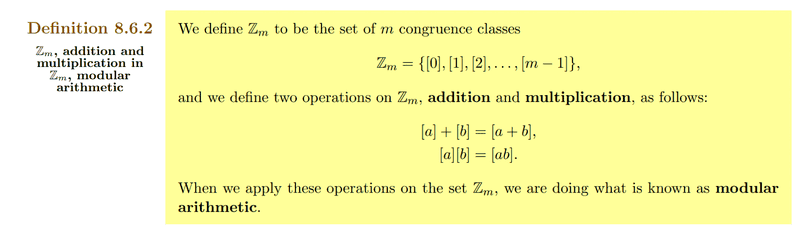# Multiplication and addition definition of congruence classes

• I
• Leo Liu
In summary, the conversation discusses the definitions of congruence classes and their operations, such as addition and multiplication. These definitions may seem arbitrary or an abuse of notation, but they are actually based on the pre-existing definitions in the integers. They also have practical applications, such as simplifying the process of solving modular equations and playing a role in cryptography. There is some overloading of notation in this context, but it can be disambiguated based on context.

#### Leo Liu

The definitions of them seem like arbitrary choices or an abuse of notation. I wonder what the reasons behind the definitions are. Thanks.PS. My instructor said such defs simplify the process of solving modular equations.

Last edited:
It's quite standard. It just means, for example if you consider ##\mathbf{Z}_4##, that\begin{align*}
 +  &=  \\
&= 
\end{align*}et cetera.

Leo Liu said:
The definitions of them seem like arbitrary choices or an abuse of notation. I wonder what the reasons behind the definitions are. Thanks.

View attachment 292853
PS. My instructor said such defs simply the process of solving modular equations.
You can view this from various perspectives. The shortest notation is, that ##\pi\, : \,\mathbb{Z}\longrightarrow \mathbb{Z}/m\mathbb{Z}## is a homomorphism of rings. This requires ##\mathbb{Z}/m\mathbb{Z}## to be a ring, i.e. that we multiply and add the remainders by division with ##m## in such a way, that adding and multiplication commutes with taking the remainders. The numbers ##\{0,1,\ldots,m-1\}## build representatives of the equivalence classes achieved by taking the remainder by division with ##m##.

More prosaic, we could say that taking the remainders is important in number theory, where we often distinguish primes which have the remainder ##1## by division with ##4## and those with remainder ##3##.

Another important example is when ##m## itself is prime. Then those calculation rules define a finite number field, i.e. we can even divide by the equivalence classes ##[a]\neq .## In case ##m=2## we get thus the basic rules for computers, or less complicated: the light switch in your room. Finite fields in general (##m## prime) play an important role in cryptography, i.e. the science of codes.

If ##m## is not prime, say ##m=p\cdot q## then we cannot divide, i.e. we have still a ring but no field. This is because ##[p]\cdot [q]=## although ##[p],[q]\neq .## An example for such a case is the analogous clock. The clock has ##m=12## for the big hand, and ##m=60## for the small hand.

•Leo Liu
Leo Liu said:
The definitions of them seem like arbitrary choices or an abuse of notation. I wonder what the reasons behind the definitions are. Thanks.

View attachment 292853
PS. My instructor said such defs simply the process of solving modular equations.
One is defining addition ("+") for congruence classes based on the pre-existing definition of addition ("+") for class members.

There is a bit of abuse of notation going on here. The same graphical symbol ("+") is used for both operations. This sort of notation abuse is sometimes called "overloading". We "overload" an operator with two possible meanings. Then we disambiguate between the meaning based on context. If one sees the "+" operator applied to two congruence classes, one knows that the class meaning is appropriate and the result will be a congruence class. If one sees the "+" operator applied to two class members, one knows that the member meaning is appropriate and the result will be a class member.

One can find this particular notational pattern used in some programming languages. For instance, in the Ada programming language, one can overload or even redefine infix operators such as "+" and "-".

Let us read through [a] + [b] = [a+b] and try to express it in words:

"If we take the congruence class containing a and "add" it to the congruence class containing b, the result is defined to be the congruence class containing a+b"

One should normally take a few moments to verify that this definition actually works as a definition.

What if, instead of "a", we took a different exemplar, say "x", of the left hand congruence class.
What if, instead of "b", we took a different exemplar, say "y" of the right hand congruence class.
Would the sum of those two exemplars have been a member of the same congruence class.

In other words, if [a] = [x] and [b] = [y] does it follow that [a+b] = [x+y]?

If so, the definition can work.
If not, the definition is broken.

Last edited:
•Leo Liu
jbriggs444 said:
There is a bit of abuse of notation going on here. The same graphical symbol ("+") is used for both operations.
It's also used for adding vectors, and matrices, and polynomials, ... and generally everything that looks like an addition in a set.

The addition and multiplication defined in the first post are derived from addition and multiplication in the integers. If we add an integer in the equivalence class [a] and an integer in the equivalence class [b] we get an integer in the equivalence class [a+b], so it makes sense to define an operation within the set of equivalence classes as well. Same for multiplication.

Edit: Fixed formatting

Last edited:
•Leo Liu and jbriggs444
ergospherical said:
It's quite standard. It just means, for example if you consider ##\mathbf{Z}_4##, that\begin{align*}
 +  &=  \\
&= 
\end{align*}et cetera.
Hey, I didn't know you were still alive!
•